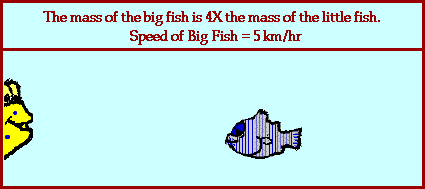## Big Fish in Motion Catches Little Fish

### Inelastic Collision

The animation below portrays the inelastic collision between a very massive fish and a less massive fish. Before the collision, the big fish is in motion with a velocity of 5 km/hr and the little fish is at rest. The big fish has four times the mass of the little fish. After the collision, both the big fish and the little fish move together with the same velocity. Collisions such as this where the two objects stick together and move with the same post-collision velocity are referred to as inelastic collisions. What is the after-collision velocity of the two fish?Collisions between objects are governed by laws of momentum and energy. When a collision occurs in an isolated system, the total momentum of the system of objects is conserved. Provided that there are no net external forces acting upon the two fish, the momentum of the big and little fish before the collision equals the momentum of the big and little fish after the collision

The mathematics of this problem is simplified by the fact that before the collision, there is only one object in motion and after the collision both objects have the same velocity. That is to say, a momentum analysis would show that all the momentum was concentrated in the big fish before the collision. And after the collision, all the momentum was the result of a single object (the combination of the big and little fish) moving at an easily predictable velocity.

The prediction of the final velocity of the two fish involves determining the ratio by which the mass which is in motion changed; and then dividing the initial velocity by that ratio. That is, if the amount of mass in motion increases by a factor of two, then the velocity would decrease by a factor of two (divide the original velocity by two). If the amount of mass in motion increases by a factor of five, then the velocity would decrease by a factor of five (divide the original velocity by five). In the case of the animation above, the amount of mass in motion increased by a factor of 5/4; a change from say 4 kg for the big fish before the collision to 5 kg for the combination of the big and little fish after the collision. (Note that I can make up any numbers for mass as long as they meet the criteria that the big fish has four times the mass as the little fish.) Since the amount of mass in motion increased by a factor of 5/4, the velocity at which that mass is in motion must decrease by a factor of 5/4. That is, the original velocity of 5 km/hr must be divided by 5/4. The result is 4 km/hr; the big and little fish move together with a velocity of 4 km/hr after the collision.

For more information on physical descriptions of motion, visit The Physics Classroom Tutorial. Detailed information is available there on the following topics:

Momentum

Momentum Conservation Principle

Isolated Systems

Momentum Conservation in Collisions